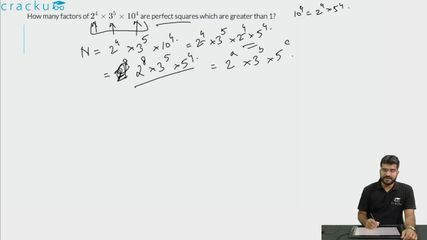Question 84

# How many factors of $$2^4 \times 3^5 \times 10^4$$ are perfect squares which are greater than 1?

Solution

$$2^4 \times 3^5 \times 10^4$$

=$$2^4 \times 3^5 \times 2^4*5^4$$

=$$2^8 \times 3^5 \times 5^4$$

For the factor to be a perfect square, the factor should be even power of the number.

In $$2^8$$, the factors which are perfect squares are $$2^0, 2^2, 2^4, 2^6, 2^8$$ = 5

Similarly, in $$3^5$$, the factors which are perfect squares are $$3^0, 3^2, 3^4$$ = 3

In $$5^4$$, the factors which are perfect squares are $$5^0, 5^2, 5^4$$ = 3

Number of perfect squares greater than 1 = 5*3*3-1

=44

### View Video Solution